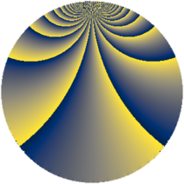# Properties

 Label 525.3.buLevel $525$ Weight $3$ Character orbit 525.bu Rep. character $\chi_{525}(37,\cdot)$ Character field $\Q(\zeta_{60})$ Dimension $1280$ Sturm bound $240$

# Related objects

## Defining parameters

 Level: $$N$$ $$=$$ $$525 = 3 \cdot 5^{2} \cdot 7$$ Weight: $$k$$ $$=$$ $$3$$ Character orbit: $$[\chi]$$ $$=$$ 525.bu (of order $$60$$ and degree $$16$$) Character conductor: $$\operatorname{cond}(\chi)$$ $$=$$ $$175$$ Character field: $$\Q(\zeta_{60})$$ Sturm bound: $$240$$

## Dimensions

The following table gives the dimensions of various subspaces of $$M_{3}(525, [\chi])$$.

Total New Old
Modular forms 2624 1280 1344
Cusp forms 2496 1280 1216
Eisenstein series 128 0 128

## Trace form

 $$1280 q - 4 q^{5} + 4 q^{7} - 24 q^{8} + O(q^{10})$$ $$1280 q - 4 q^{5} + 4 q^{7} - 24 q^{8} + 16 q^{10} + 48 q^{15} - 640 q^{16} + 224 q^{17} - 64 q^{22} - 32 q^{23} + 4 q^{25} + 140 q^{28} + 400 q^{29} - 192 q^{30} + 48 q^{32} + 72 q^{33} - 296 q^{35} - 1920 q^{36} + 28 q^{37} - 592 q^{38} - 164 q^{40} + 12 q^{42} + 616 q^{43} - 412 q^{47} + 288 q^{48} - 288 q^{50} + 152 q^{52} - 600 q^{53} + 8 q^{55} + 192 q^{57} - 56 q^{58} + 800 q^{59} + 180 q^{60} + 912 q^{62} + 84 q^{63} - 1800 q^{64} - 20 q^{65} + 368 q^{67} + 492 q^{68} - 96 q^{70} - 36 q^{72} + 316 q^{73} - 96 q^{75} - 844 q^{77} - 624 q^{78} - 908 q^{80} - 1440 q^{81} + 84 q^{82} + 1152 q^{83} - 2400 q^{84} + 536 q^{85} - 108 q^{87} + 948 q^{88} + 864 q^{90} + 1704 q^{92} + 576 q^{93} + 380 q^{95} + 208 q^{97} - 832 q^{98} + O(q^{100})$$

## Decomposition of $$S_{3}^{\mathrm{new}}(525, [\chi])$$ into newform subspaces

The newforms in this space have not yet been added to the LMFDB.

## Decomposition of $$S_{3}^{\mathrm{old}}(525, [\chi])$$ into lower level spaces

$$S_{3}^{\mathrm{old}}(525, [\chi]) \cong$$ $$S_{3}^{\mathrm{new}}(175, [\chi])$$$$^{\oplus 2}$$